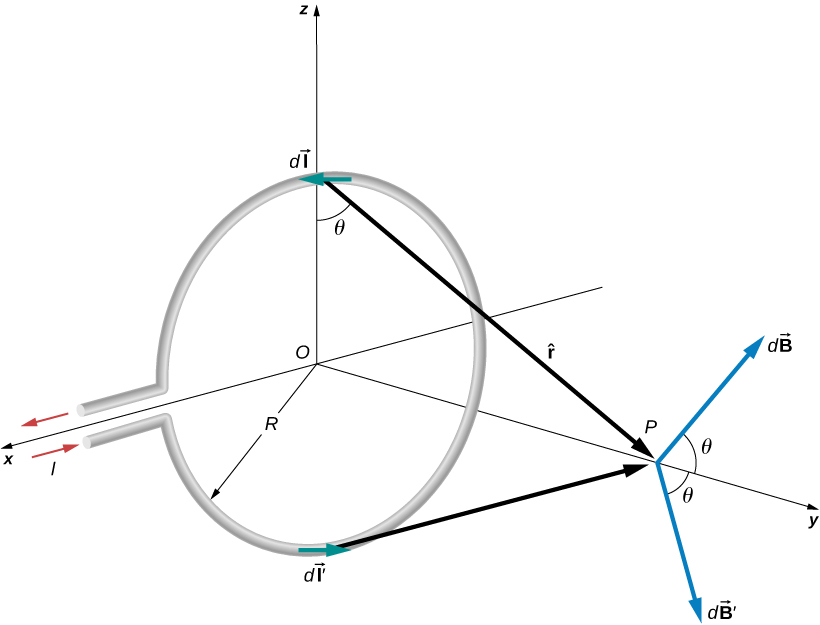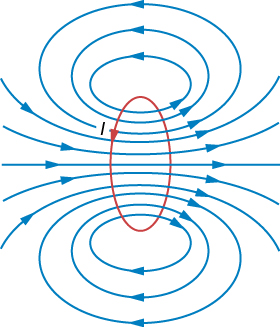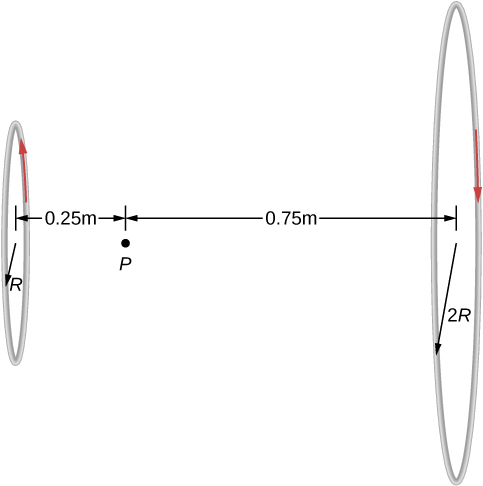# 12.4 Magnetic field of a current loop

 Page 1 / 1
By the end of this section, you will be able to:
• Explain how the Biot-Savart law is used to determine the magnetic field due to a current in a loop of wire at a point along a line perpendicular to thep lane of the loop.
• Determine the magnetic field of an arc of current.

The circular loop of [link] has a radius R , carries a current I , and lies in the xz -plane. What is the magnetic field due to the current at an arbitrary point P along the axis of the loop?Determining the magnetic field at point P along the axis of a current-carrying loop of wire.

We can use the Biot-Savart law to find the magnetic field due to a current. We first consider arbitrary segments on opposite sides of the loop to qualitatively show by the vector results that the net magnetic field direction is along the central axis from the loop. From there, we can use the Biot-Savart law to derive the expression for magnetic field.

Let P be a distance y from the center of the loop. From the right-hand rule, the magnetic field $d\stackrel{\to }{B}$ at P , produced by the current element $I\phantom{\rule{0.2em}{0ex}}d\stackrel{\to }{l},$ is directed at an angle $\theta$ above the y -axis as shown. Since $d\stackrel{\to }{l}$ is parallel along the x -axis and $\stackrel{^}{r}$ is in the yz -plane, the two vectors are perpendicular, so we have

$dB=\frac{{\mu }_{0}}{4\pi }\phantom{\rule{0.2em}{0ex}}\frac{I\phantom{\rule{0.2em}{0ex}}dl\phantom{\rule{0.2em}{0ex}}\mathrm{sin}\phantom{\rule{0.1em}{0ex}}\theta }{{r}^{2}}=\frac{{\mu }_{0}}{4\pi }\phantom{\rule{0.2em}{0ex}}\frac{I\phantom{\rule{0.2em}{0ex}}dl}{{y}^{2}+{R}^{2}}$

where we have used ${r}^{2}={y}^{2}+{R}^{2}.$

Now consider the magnetic field $d{\stackrel{\to }{B}}^{\prime }$ due to the current element $I\phantom{\rule{0.2em}{0ex}}d{\stackrel{\to }{l}}^{\prime },$ which is directly opposite $I\phantom{\rule{0.2em}{0ex}}d\stackrel{\to }{l}$ on the loop. The magnitude of $d{\stackrel{\to }{B}}^{\prime }$ is also given by [link] , but it is directed at an angle $\theta$ below the y -axis. The components of $d\stackrel{\to }{B}$ and $d{\stackrel{\to }{B}}^{\prime }$ perpendicular to the y -axis therefore cancel, and in calculating the net magnetic field, only the components along the y -axis need to be considered. The components perpendicular to the axis of the loop sum to zero in pairs. Hence at point P :

$\stackrel{\to }{B}=\stackrel{^}{j}\underset{\text{loop}}{\int }dB\phantom{\rule{0.2em}{0ex}}\mathrm{cos}\phantom{\rule{0.1em}{0ex}}\theta =\stackrel{^}{j}\frac{{\mu }_{0}I}{4\pi }\underset{\text{loop}}{\int }\frac{\mathrm{cos}\phantom{\rule{0.1em}{0ex}}\theta \phantom{\rule{0.2em}{0ex}}dl}{{y}^{2}+{R}^{2}}.$

For all elements $d\stackrel{\to }{l}$ on the wire, y , R , and $\mathrm{cos}\phantom{\rule{0.1em}{0ex}}\theta$ are constant and are related by

$\mathrm{cos}\phantom{\rule{0.1em}{0ex}}\theta =\frac{R}{\sqrt{{y}^{2}+{R}^{2}}}.$

Now from [link] , the magnetic field at P is

$\stackrel{\to }{B}=\stackrel{^}{j}\frac{{\mu }_{0}IR}{4\pi {\left({y}^{2}+{R}^{2}\right)}^{3\text{/}2}}\underset{\text{loop}}{\int }dl=\frac{{\mu }_{0}I{R}^{2}}{2{\left({y}^{2}+{R}^{2}\right)}^{3\text{/}2}}\stackrel{^}{j}$

where we have used $\underset{\text{loop}}{\int }dl=2\pi R.$ As discussed in the previous chapter, the closed current loop is a magnetic dipole of moment $\stackrel{\to }{\mu }=IA\stackrel{^}{n}.$ For this example, $A=\pi {R}^{2}$ and $\stackrel{^}{n}=\stackrel{^}{j},$ so the magnetic field at P can also be written as

$\stackrel{\to }{B}=\frac{{\mu }_{0}\mu \stackrel{^}{j}}{2\pi {\left({y}^{2}+{R}^{2}\right)}^{3\text{/}2}}.$

By setting $y=0$ in [link] , we obtain the magnetic field at the center of the loop:

$\stackrel{\to }{B}=\frac{{\mu }_{0}I}{2R}\stackrel{^}{j}.$

This equation becomes $B={\mu }_{0}nI/\left(2R\right)$ for a flat coil of n loops per length. It can also be expressed as

$\stackrel{\to }{B}=\frac{{\mu }_{0}\stackrel{\to }{\mu }}{2\pi {R}^{3}}.$

If we consider $y\gg R$ in [link] , the expression reduces to an expression known as the magnetic field from a dipole:

$\stackrel{\to }{B}=\frac{{\mu }_{0}\stackrel{\to }{\mu }}{2\pi {y}^{3}}.$

The calculation of the magnetic field due to the circular current loop at points off-axis requires rather complex mathematics, so we’ll just look at the results. The magnetic field lines are shaped as shown in [link] . Notice that one field line follows the axis of the loop. This is the field line we just found. Also, very close to the wire, the field lines are almost circular, like the lines of a long straight wire.Sketch of the magnetic field lines of a circular current loop.

## Magnetic field between two loops

Two loops of wire carry the same current of 10 mA, but flow in opposite directions as seen in [link] . One loop is measured to have a radius of $R=50\phantom{\rule{0.2em}{0ex}}\text{cm}$ while the other loop has a radius of $2R=100\phantom{\rule{0.2em}{0ex}}\text{cm}.$ The distance from the first loop to the point where the magnetic field is measured is 0.25 m, and the distance from that point to the second loop is 0.75 m. What is the magnitude of the net magnetic field at point P ?Two loops of different radii have the same current but flowing in opposite directions. The magnetic field at point P is measured to be zero.

## Strategy

The magnetic field at point P has been determined in [link] . Since the currents are flowing in opposite directions, the net magnetic field is the difference between the two fields generated by the coils. Using the given quantities in the problem, the net magnetic field is then calculated.

## Solution

Solving for the net magnetic field using [link] and the given quantities in the problem yields

$\begin{array}{ccc}\hfill B& =\hfill & \frac{{\mu }_{0}I{R}_{1}{}^{2}}{2{\left({y}_{1}{}^{2}+{R}_{1}{}^{2}\right)}^{3\text{/}2}}-\phantom{\rule{0.05em}{0ex}}\frac{{\mu }_{0}I{R}_{2}{}^{2}}{2{\left({y}_{2}{}^{2}+{R}_{2}{}^{2}\right)}^{3\text{/}2}}\hfill \\ \hfill B& =\hfill & \frac{\left(4\pi \phantom{\rule{0.2em}{0ex}}×\phantom{\rule{0.2em}{0ex}}{10}^{\text{−7}}\text{T}\cdot \text{m/A}\right)\left(0.010\phantom{\rule{0.2em}{0ex}}\text{A}\right){\left(0.5\phantom{\rule{0.2em}{0ex}}\text{m}\right)}^{2}}{2{\left({\left(0.25\phantom{\rule{0.2em}{0ex}}\text{m}\right)}^{2}+{\left(0.5\phantom{\rule{0.2em}{0ex}}\text{m}\right)}^{2}\right)}^{3\text{/}2}}-\phantom{\rule{0.05em}{0ex}}\frac{\left(4\pi \phantom{\rule{0.2em}{0ex}}×\phantom{\rule{0.2em}{0ex}}{10}^{\text{−7}}\text{T}\cdot \text{m/A}\right)\left(0.010\phantom{\rule{0.2em}{0ex}}\text{A}\right){\left(1.0\phantom{\rule{0.2em}{0ex}}\text{m}\right)}^{2}}{2{\left({\left(0.75\phantom{\rule{0.2em}{0ex}}\text{m}\right)}^{2}+{\left(1.0\phantom{\rule{0.2em}{0ex}}\text{m}\right)}^{2}\right)}^{3\text{/}2}}\hfill \\ \hfill B& =\hfill & 5.77\phantom{\rule{0.2em}{0ex}}×\phantom{\rule{0.2em}{0ex}}{10}^{\text{−9}}\text{T}\phantom{\rule{0.2em}{0ex}}\text{to the right}.\hfill \end{array}$

## Significance

Helmholtz coils typically have loops with equal radii with current flowing in the same direction to have a strong uniform field at the midpoint between the loops. A similar application of the magnetic field distribution created by Helmholtz coils is found in a magnetic bottle that can temporarily trap charged particles. See Magnetic Forces and Fields for a discussion on this.

Check Your Understanding Using [link] , at what distance would you have to move the first coil to have zero measurable magnetic field at point P ?

0.608 meters

## Summary

• The magnetic field strength at the center of a circular loop is given by $B=\frac{{\mu }_{0}I}{2R}\phantom{\rule{0.2em}{0ex}}\text{(at center of loop)},$ where R is the radius of the loop. RHR-2 gives the direction of the field about the loop.

## Conceptual questions

Is the magnetic field of a current loop uniform?

What happens to the length of a suspended spring when a current passes through it?

The spring reduces in length since each coil with have a north pole-produced magnetic field next to a south pole of the next coil.

Two concentric circular wires with different diameters carry currents in the same direction. Describe the force on the inner wire.

## Problems

When the current through a circular loop is 6.0 A, the magnetic field at its center is $2.0\phantom{\rule{0.2em}{0ex}}×\phantom{\rule{0.2em}{0ex}}{10}^{\text{−4}}\text{T}.$ What is the radius of the loop?

0.019 m

How many turns must be wound on a flat, circular coil of radius 20 cm in order to produce a magnetic field of magnitude $4.0\phantom{\rule{0.2em}{0ex}}×\phantom{\rule{0.2em}{0ex}}{10}^{\text{−5}}\text{T}$ at the center of the coil when the current through it is 0.85 A?

A flat, circular loop has 20 turns. The radius of the loop is 10.0 cm and the current through the wire is 0.50 A. Determine the magnitude of the magnetic field at the center of the loop.

$6.28\phantom{\rule{0.2em}{0ex}}×\phantom{\rule{0.2em}{0ex}}{10}^{\text{−5}}\text{T}$

A circular loop of radius R carries a current I . At what distance along the axis of the loop is the magnetic field one-half its value at the center of the loop?

Two flat, circular coils, each with a radius R and wound with N turns, are mounted along the same axis so that they are parallel a distance d apart. What is the magnetic field at the midpoint of the common axis if a current I flows in the same direction through each coil?

$B=\frac{{\mu }_{o}I{R}^{2}}{{\left({\left(\frac{d}{2}\right)}^{2}+{R}^{2}\right)}^{3\text{/}2}}$

For the coils in the preceding problem, what is the magnetic field at the center of either coil?

Is it possible to find the magnetic field of a circular loop at the centre by using ampere's law?
Is it possible to find the magnetic field of a circular loop at it's centre?
yes
Brother
The density of a gas of relative molecular mass 28 at a certain temperature is 0.90 K kgmcube.The root mean square speed of the gas molecules at that temperature is 602ms.Assuming that the rate of diffusion of a gas in inversely proportional to the square root of its density,calculate the density of
A hot liquid at 80degree Celsius is added to 600g of the same liquid originally at 10 degree Celsius. when the mixture reaches 30 degree Celsius, what will be the total mass of the liquid?
what is electrostatics
Study of charges which are at rest
himanshu
Explain Kinematics
Two equal positive charges are repelling each other. The force on the charge on the left is 3.0 Newtons. Using your notes on Coulomb's law, and the forces acting on each of the charges, what is the force on the charge on the right?
Using the same two positive charges, the left positive charge is increased so that its charge is 4 times LARGER than the charge on the right. Using your notes on Coulomb's law and changes to the charge, once the charge is increased, what is the new force of repulsion between the two positive charges?
Nya
A mass 'm' is attached to a spring oscillates every 5 second. If the mass is increased by a 5 kg, the period increases by 3 second. Find its initial mass 'm'
a hot water tank containing 50,000g of water is heated by an electric immersion heater rated at 3kilowatt,240volt, calculate the current
what is charge
product of current and time
Jaffar
Why always amber gain electrons and fur loose electrons? Why the opposite doesn't happen?
A closely wound search coil has an area of 4cm^2,1000 turns and a resistance of 40ohm. It is connected to a ballistic galvanometer whose resistance is 24 ohm. When coil is rotated from a position parallel to uniform magnetic field to one perpendicular to field,the galvanometer indicates a charge
Using Kirchhoff's rules, when choosing your loops, can you choose a loop that doesn't have a voltage?
how was the check your understand 12.7 solved?
Who is ISSAAC NEWTON
he's the father of 3 newton law
Hawi
he is Chris Issaac's father :)
Ethem
how to name covalent bond
Who is ALEXANDER BELL
LOAKBy OpenStaxBy Brooke DelaneyBy Madison ChristianBy Stephen VoronBy OpenStaxBy Gerr ZenBy Christine ZeelieBy John GabrieliBy JavaChamp TeamBy Vanessa Soledad